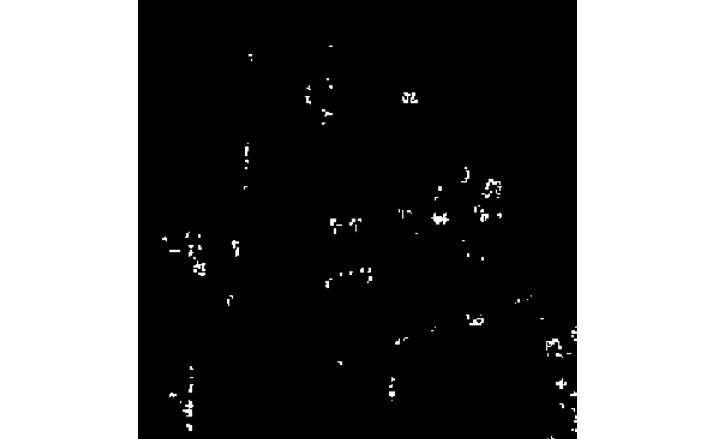# 八、高通过滤器和特征检测

• 探索高通过滤器
• 使用 Canny 边缘检测器
• 使用霍夫变换查找圆和直线
• 哈里斯角点检测

# 探索高通过滤器

OpenCV 有许多库函数，可实现高通过滤器。 我们将研究如何使用Laplacian()Sobel()Scharr()函数。

https://www.tutorialspoint.com/dip/Sobel_operator.htm

https://www.tutorialspoint.com/dip/Laplacian_Operator.htm

• src：这是要在其中检测边缘的源图像的参数。
• ddepth：这是用于确定目标图像深度的参数。-1表示源图像和目标图像具有相同的深度。 OpenCV 提供的高通滤波函数支持源图像和目标图像深度的以下组合：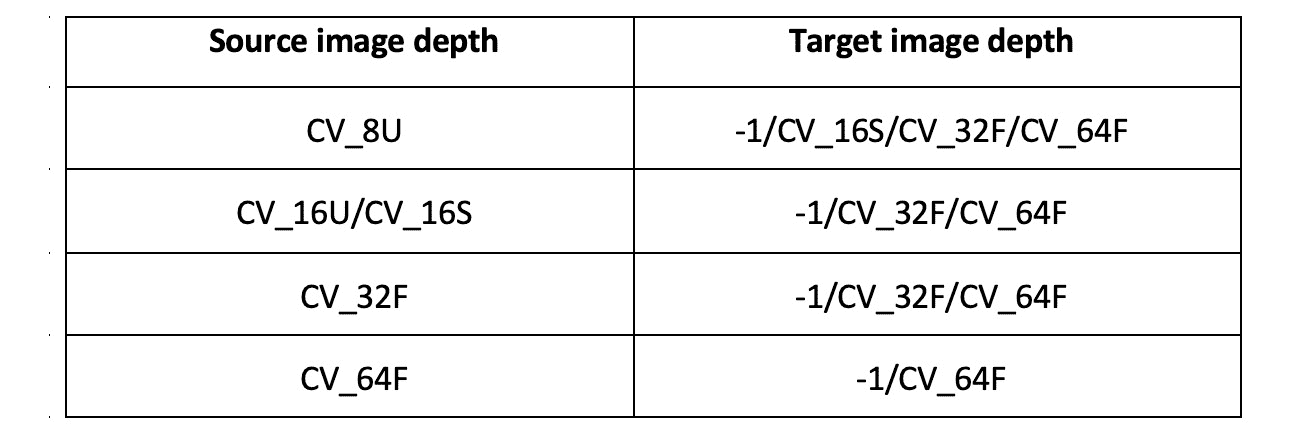• dx：这是X的导数的顺序（Laplacian()不需要）。
• dy：这是Y的导数的顺序（Laplacian()不需要）。
• ksize：这是核矩阵的大小（Sobel()函数或Laplacian()函数的正奇数，可以是1357Scharr()不需要）。
• scale：这是标度，是可选的。 这是计算的拉普拉斯算子值的可选比例的因素。 默认情况下不应用缩放。
• delta：这是增量的值。 这是一个可选常数，并添加到最终输出中。
• borderType：这是用于对位于边界处的像素进行像素外推的方法。

import cv2
import matplotlib.pyplot as plt
laplacian = cv2.Laplacian(img, ddepth=cv2.CV_32F, ksize=17,
scale=1, delta=0,
borderType=cv2.BORDER_DEFAULT)
sobel = cv2.Sobel(img, ddepth=cv2.CV_32F, dx=1, dy=0,
ksize=11, scale=1, delta=0,
borderType=cv2.BORDER_DEFAULT)
scharr = cv2.Scharr(img, ddepth=cv2.CV_32F, dx=1, dy=0,
scale=1, delta=0,
borderType=cv2.BORDER_DEFAULT)
images=[img, laplacian, sobel, scharr]
titles=['Original', 'Laplacian', 'Sobel', 'Scharr']
for i in range(4):
plt.subplot(2, 2, i+1)s
plt.imshow(images[i], cmap = 'gray')
plt.title(titles[i])
plt.axis('off')
plt.show()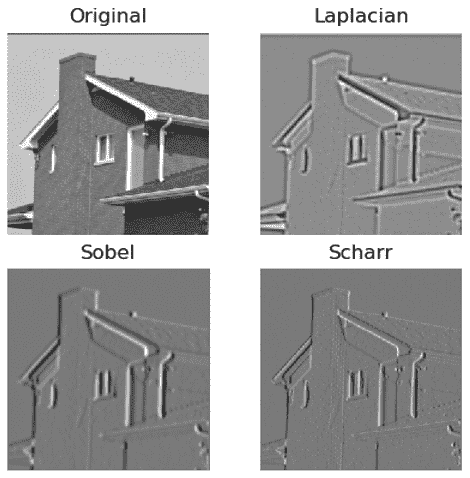import RPi.GPIO as GPIO
import cv2
x = 0
y = 1
cap = cv2.VideoCapture(0)
GPIO.setmode(GPIO.BOARD)
GPIO.setwarnings(False)
button1 = 7
button2 = 11
GPIO.setup(button1, GPIO.IN, GPIO.PUD_UP)
GPIO.setup(button2, GPIO.IN, GPIO.PUD_UP)
while True:
print(x, y)
button1_state = GPIO.input(button1)
if button1_state == GPIO.LOW:
x = 0
y = 1
button2_state = GPIO.input(button2)
if button2_state == GPIO.LOW:
x = 1
y = 0


    output = cv2.Scharr(frame, ddepth=cv2.CV_32F,
dx=x, dy=y,
scale=1, delta=0,
borderType=cv2.BORDER_DEFAULT)
cv2.imshow('Salt and pepper Noise App', output)
if cv2.waitKey(1) == 27:
break
cap.release()
cv2.destroyAllWindows()


import cv2
cap = cv2.VideoCapture(0)
while True:
output1 = cv2.Scharr(frame, ddepth=cv2.CV_32F,
dx=0, dy=1,
scale=1, delta=0,
borderType=cv2.BORDER_DEFAULT)


    output2 = cv2.Scharr(frame, ddepth=cv2.CV_32F,
dx=1, dy=0,
scale=1, delta=0,
borderType=cv2.BORDER_DEFAULT)
if cv2.waitKey(1) == 27:
break
cap.release()
cv2.destroyAllWindows()


# 使用 Canny 边缘检测器

Canny 边缘检测算法由 John Canny 开发。 Canny 的算法大量使用高通过滤器的概念。 它具有多个步骤。

OpenCV 具有cv2.Canny()函数，提供了 Canny 算法。 以下是该算法的步骤：

1. 将具有5 x 5像素大小的高斯核应用于输入图像以消除任何噪声。
2. 然后，我们计算滤波图像强度的梯度。 我们可以在此步骤中使用 L1 或 L2 范数。
3. 然后，我们应用非最大抑制，并为可能的边缘集确定候选者。
4. 最后一步是磁滞的操作。 我们根据传递给图像的阈值最终确定边缘。

注意：

您可以在这个页面这个页面上详细了解 L1 和 L2 规范以及非最大抑制。

• img：我们需要检测边缘的输入源图像。
• threshold1：阈值的下限。
• threshold2：阈值的上限。
• L2gradient：如果此值为True，则该函数使用 L2 范数来计算边集，这更精确，但计算量大。 如果为False，则使用 L1 范数来计算边集，这需要较少的计算，但准确率较低。

import cv2
import matplotlib.pyplot as plt
edges1 = cv2.Canny(img, 50, 300, L2gradient=False)
edges2 = cv2.Canny(img, 100, 150, L2gradient=True)
images = [img, edges1, edges2]
for i in range(3):
plt.subplot(1, 3, i+1)
plt.imshow(images[i], cmap = 'gray')
plt.title(titles[i])
plt.axis('off')
plt.show()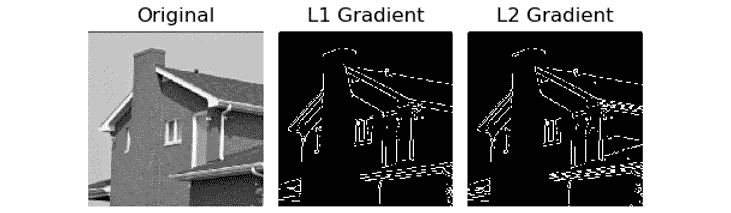import cv2
cv2.namedWindow('Canny')
def empty(z):
pass
cv2.createTrackbar('Threshold 1', 'Canny', 50, 100, empty)
cv2.createTrackbar('Threshold 2', 'Canny', 150, 300, empty)
while(True):
l1 = cv2.getTrackbarPos('Threshold 1', 'Canny')
l2 = cv2.getTrackbarPos('Threshold 2', 'Canny')
output = cv2.Canny(img, l1, l2, L2gradient=False)
cv2.imshow('Canny', output)
if cv2.waitKey(1) == 27:
break
cv2.destroyAllWindows()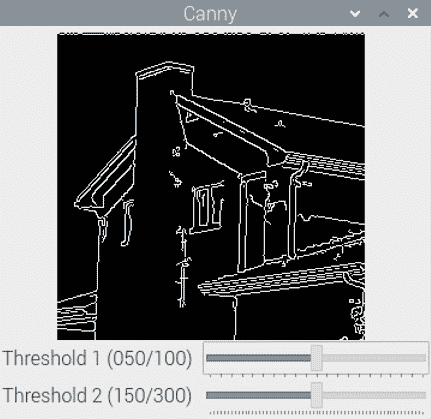## 使用霍夫变换查找圆和直线

OpenCV 提供了cv2.HoughCircles()函数，用于使用霍夫方法检测图像中的圆。 这将返回检测到的圆的中心和半径。 它接受图像，（cv2.HOUGH_GRADIENT）检测方法，分辨率的反比，要检测的圆心之间的最小距离，内部使用的 Canny 方法的最高阈值 ，累加器的阈值以及要检测的圆的最大和最小距离。

import cv2
cap = cv2.VideoCapture(0)
while (True):
grey = cv2.cvtColor(frame, cv2.COLOR_BGR2GRAY)
blur = cv2.blur(grey, (5, 5))
circles = cv2.HoughCircles(blur,
dp=1, minDist=200,
param1=50, param2=13,
if circles is not None:
for i in circles [0,:]:
cv2.circle(frame, (i, i), i, (0, 255, 0), 2)
cv2.circle(frame, (i, i), 2, (0, 0, 255), 3)
cv2.imshow('Detected', frame)
if cv2.waitKey(1) == 27:
break
cv2.destroyAllWindows()
cap.release()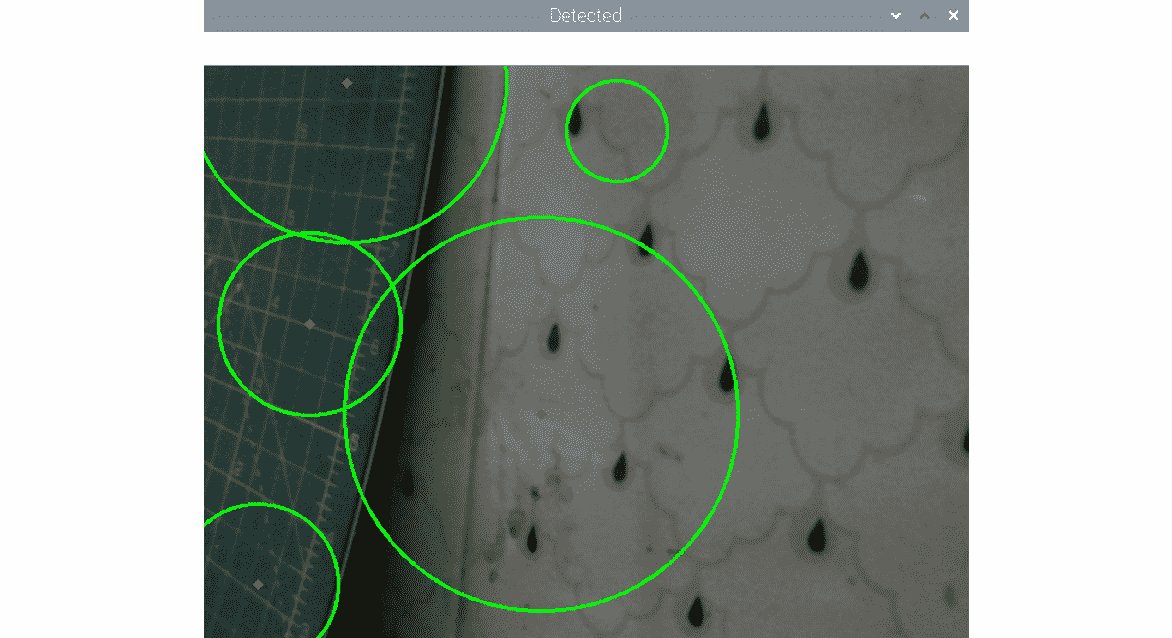OpenCV cv2.HoughLines()函数检测图像中的线。 它接受灰度图像，ρ值（累加器的距离精度），θ（累加器的角度精度）和累加器阈值参数作为参数。 我们将通过实时 USB 网络摄像头视频提要进行演示。 返回的输出为极坐标格式，必须在可视化之前将其转换为X/Y坐标系：

import numpy as np
import cv2
cap = cv2.VideoCapture(0)
while True:
gray = cv2.cvtColor(img, cv2.COLOR_BGR2GRAY)
edges = cv2.Canny(gray, 50, 250, apertureSize=5,
lines = cv2.HoughLines(edges, 1, np.pi/180, 200)
if lines is not None:
for rho,theta in lines:
a = np.cos(theta)
b = np.sin(theta)
x0 = a*rho
y0 = b*rho
pts1 = (int(x0 + 1000*(-b)), int(y0 + 1000*(a)))
pts2 = (int(x0 - 1000*(-b)), int(y0 - 1000*(a)))
cv2.line(img, pts1, pts2, (0, 0, 255), 2)
cv2.imshow('Detected Lines', img)
if cv2.waitKey(1) == 27:
break
cv2.destroyAllWindows()
cap.release()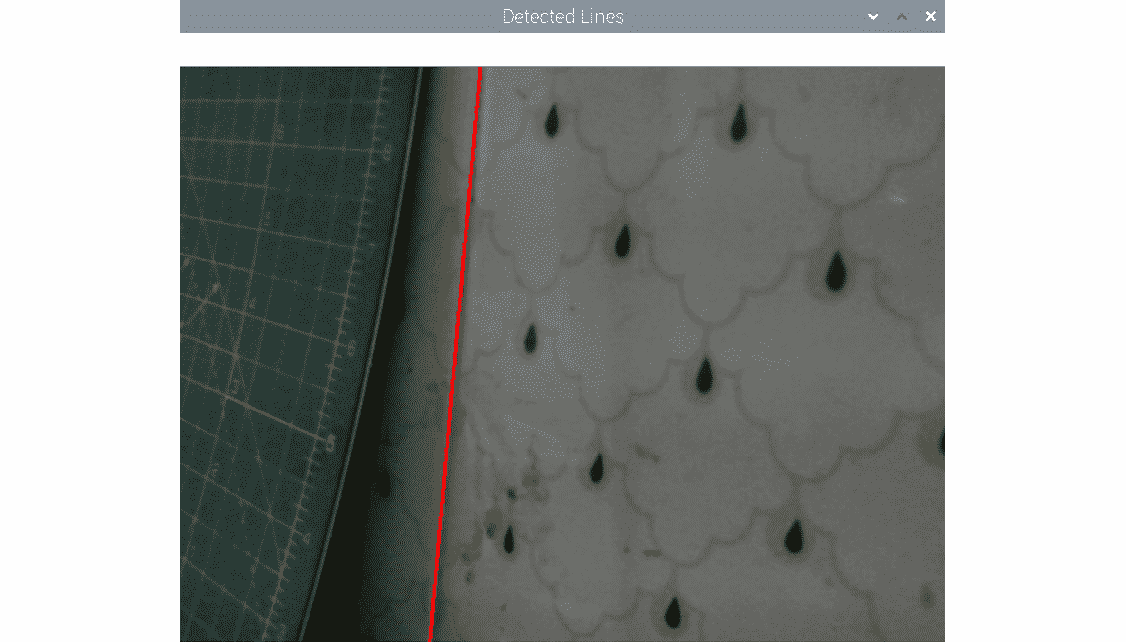# 哈里斯角点检测

OpenCV 具有cv2.cornerHarris()函数，用于检测角。 其参数如下：

• img：输入图像，必须为灰度并且具有float32类型。
• blockSize：这是考虑进行角点检测的邻域的大小。
• ksize：使用的 Sobel 导数的光圈参数。
• k：等式中使用的自由哈里斯检测器参数。

import cv2
import numpy as np
import matplotlib.pyplot as plt
img = np.float32(img)
dst = cv2.cornerHarris(img, 2, 3, 0.04)
ret, dst = cv2.threshold(dst, 0.01*dst.max(), 255, 0)
dst = np.uint8(dst)
plt.imshow(dst, cmap='gray')
plt.axis('off')
plt.show()